Maths-
General
Easy

Question

# Let a,b,c such that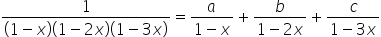then ,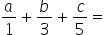?

##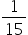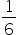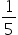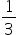Hint:

## The correct answer is:### Given :Taking LCM on RHS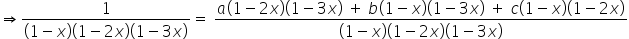Simplifying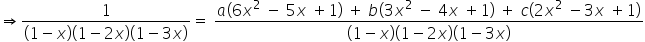Cancelling denominators on both side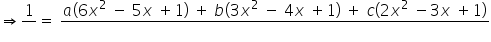Further simplifying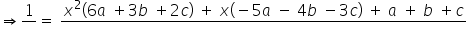Equating coefficients by comparing LHS and RHSMultiplying by 5 in equation 3 and subtracting equation 2 from it, we getMultiplying by 6 in equation 3 and subtracting equation 1 from it, we getMultiplying by 2 in equation 4 and subtracting equation 5 from it, we getMultiplying by 6 in equation 3 and subtracting equation 1 from it, we get2b + 4c = 10-3b + 4c = 6______________- b = 4b = -4Substituting value of b, we get c = 9/2 and a = 1/2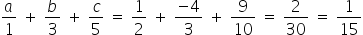#### With Turito Foundation.#### Get an Expert Advice From Turito.